Latest Banking jobs   »

# Reasoning Ability Quiz For NABARD Grade A 2022- 2nd August

Directions (1-5): Study the following information carefully and answer the questions given below:
In a certain code language:
‘Destructive epidemic around world’ is coded as ‘pine fine nine wine’
‘World extreme economic recession’ is coded as ‘mine vine sine wine’
‘Epidemic risks economic failure’ is coded as ‘nine tine vine bine’
‘Man risks destructive recession’ is coded as ‘pine dine tine sine’

Q1. What is the code of “recession” as per the given code language?
(a) mine
(b) sine
(c) dine
(d) wine
(e) None of these

Q2. What may be the possible code of “destructive cyclone” as per the given code language?
(a) mine fine
(b) sine pine
(c) pine line
(d) wine sine
(e) mine pine

Q3. What is the code of “economic risks” as per the given code language?
(a) vine tine
(b) sine vine
(c) dine tine
(d) wine dine
(e) None of these

Q4. Which of the following word is coded as “nine” as per the given code language?
(a) world
(b) recession
(c) extreme
(d) epidemic
(e) None of these

Q5. What is the code of “extreme” as per the given code language?
(a) sine
(b) mine
(c) dine
(d) wine
(e) None of these

Directions (6-10): Study the following information carefully and answer the questions given below:
In a certain code language:
‘China America conducts meeting’ is coded as ‘x5b z7b x4t n7h’
‘Online session for students’ is coded as ‘l3f h7o u3s h4t’
‘Masks sale growing fast’ is coded as ‘n5t h2f t7h u2u’
‘School and college closed’ is coded as ‘h3m z3e x7f x3e’

Q6. What is the code of ‘escape’ as per the given code language?
(a) v6f
(b) v3f
(c) f6v
(d) f3v
(e) None of these

Q7. What is the code of ‘migrant worker’ as per the given code language?
(a) n7u d3s
(b) m7u d6s
(c) n8u w3r
(d) m8t w6s
(e) None of these

Q8. Which of the following word is coded as ‘t5t’ as per the given code language?
(a) Gathering
(b) Greetings
(c) Terrible
(d) Transport
(e) Guidelines

Q9. What is the code of ‘immunity’ as per the given code language?
(a) r8z
(b) r4y
(c) i4z
(d) i8y
(e) None of these

Q10. Which of the following pair of words is coded as ‘self isolation’ as per the given code language?
(a) h2g i9o
(b) h4g i9o
(c) h2g r9o
(d) s4g r9n
(e) s2g r9n

Directions (11-15): Study the following information carefully and answer the questions given below:
In a certain code language:
‘Hospitals provide proper facilities’ is coded as ‘yu ki fs bv’
‘Proper study guidance important’ is coded as ‘bv at zx pe’
‘Hospitals facilities not important’ is coded as ‘zx yu fs gf’
‘facilities guidance where needed’ is coded as ‘fs at hw dn’

Q11. What is the code of “hospital” as per the given code language?
(a) yu
(b) ki
(c) Either(a)or(b)
(d) hw
(e) None of these

Q12. What is the code of “important” as per the given code language?
(a) at
(b) zx
(c) gf
(d) pe
(e) None of these

Q13. What is the code of “guidance needed” as per the given code language?
(a) at pe
(b) at dn
(c) hw at
(d) Either (a)or(b)
(e) Either (b)or(c)

Q14.Which of the following word is coded as ‘bv’ per the given code language?
(a) provide
(b) study
(c) proper
(d) not
(e) None of these

Q15. What may the possible code for ‘provide help’ as per the given code language?
(a) ki bv
(b) bv zx
(c) zx hp
(d) ki hp
(e) ki yu

Solutions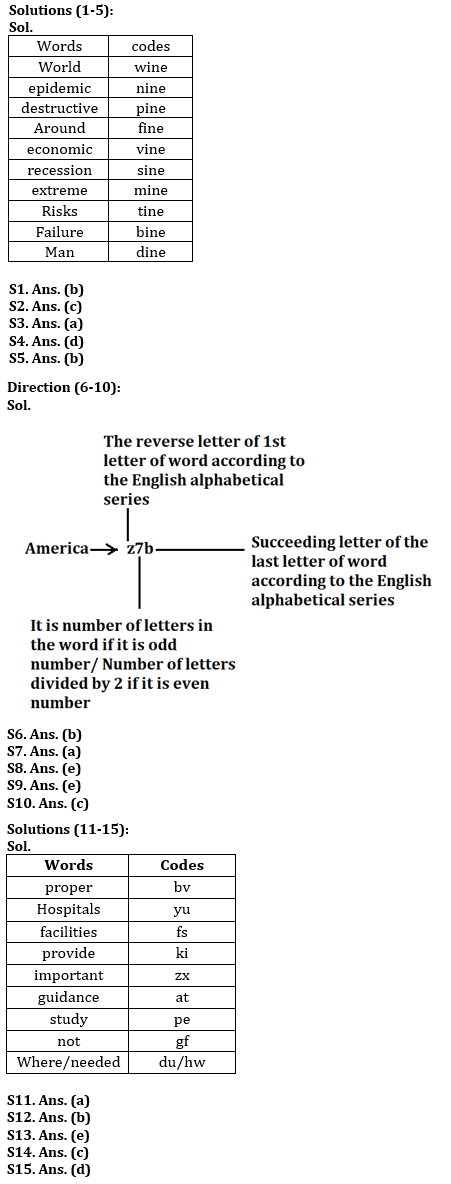#### Congratulations!Incorrect details? Fill the form again here

•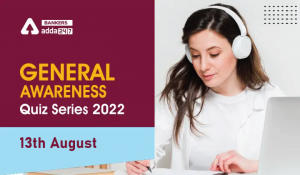General Awareness Quiz Series 2022: 13th...
•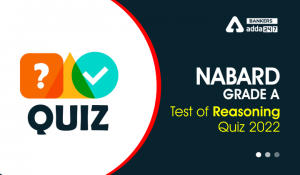Reasoning Ability Quiz For NABARD Grade ...
•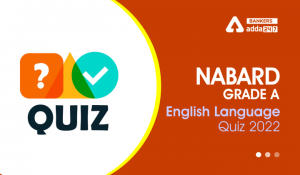English Quizzes For NABARD Grade A 2022-...
•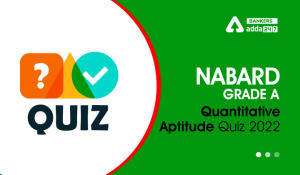Quantitative Aptitude Quiz For NABARD Gr...
•Langya Virus Found in China: Spread &...
•Reasoning Ability Quiz For IBPS RRB PO C...